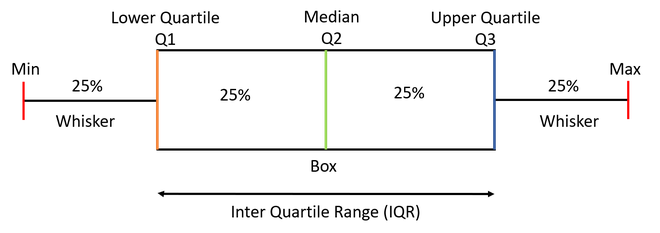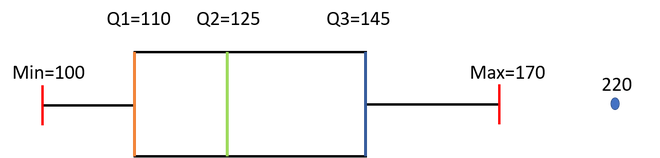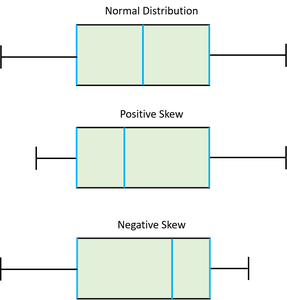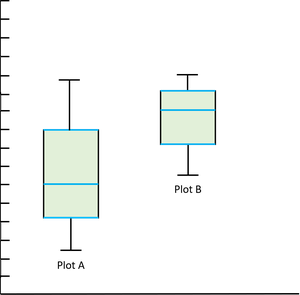# Box Plot

• Last Updated : 22 Jan, 2021

Box Plot: It is a type of chart that depicts a group of numerical data through their quartiles. It is a simple way to visualize the shape of our data. It makes comparing characteristics of data between categories very easy.

1. Understanding the components of a box plot
2. How to create a box plot
3. Uses of a Box Plot
4. How to compare box plots

Let’s proceed further step by step

1) Understanding the components of a box plot

A box plot gives a five-number summary of a set of data which is-

• Minimum – It is the minimum value in the dataset excluding the outliers
• First Quartile (Q1) – 25% of the data lies below the First (lower) Quartile.
• Median (Q2) – It is the mid-point of the dataset. Half of the values lie below it and half above.
• Third Quartile (Q3) – 75% of the data lies below the Third (Upper) Quartile.
• Maximum – It is the maximum value in the dataset excluding the outliers.Note: The box plot shown in the above diagram is a perfect plot with no skewness. The plots can have skewness and the median might not be at the center of the box.

The area inside the box (50% of the data) is known as the Inter Quartile Range. The IQR is calculated as –

`IQR = Q3-Q1`

Outliers are the data points below and above the lower and upper limit. The lower and upper limit is calculated as –

```Lower Limit = Q1 - 1.5*IQR
Upper Limit = Q3 + 1.5*IQR```

The values below and above these limits are considered outliers and the minimum and maximum values are calculated from the points which lie under the lower and upper limit.

2) How to create a box plot

Let us take a sample data to understand how to create a box plot.

Here are the runs scored by a cricket team in a league of 12 matches – 100,120,110,150,110,140,130,170,120,220,140,110.

To draw a box plot for the given data first we need to arrange the data in ascending order and then find the minimum, first quartile, median, third quartile and the maximum.

```Ascending Order -
100,110,110,110,120,120,130,140,140,150,170,220

Median (Q2) = (120+130)/2 = 125   ; Since there were even values```

To find the First Quartile we take the first six values and find their median.

`Q1 = (110+110)/2 = 110`

For the Third Quartile, we take the next six and find their median.

`Q3 = (140+150)/2 = 145`

Note: If the total number of values is odd then we exclude the Median while calculating Q1 and Q3. Here since there were two central values we included them.

Now, we need to calculate the Inter Quartile Range.

`IQR = Q3-Q1 = 145-110 = 35`

We can now calculate the Upper and Lower Limits to find the minimum and maximum values and also the outliers if any.

```Lower Limit = Q1-1.5*IQR = 110-1.5*35 = 57.5
Upper Limit = Q3+1.5*IQR = 145+1.5*35 = 197.5```

So the minimum and maximum between the range [57.5,197.5] for our given data are –

```Minimum = 100
Maximum = 170```

The outliers which are outside this range are –

`Outliers = 220`

Now we have all the information, so we can draw the box plot which is as below-We can see from the diagram that the Median is not exactly at the centre of the box and one whisker is longer than the other. We also have one Outlier.

3) Uses of a Box Plot

• Box plots provide a visual summary of the data with which we can quickly identify the average value of the data, how dispersed the data is, whether the data is skewed or not (skewness).
• The Median gives you the average value of the data.
• Box Plots shows Skewness of the data-
```a) If the Median is at the center of the Box and the whiskers are almost the
same on both the ends then the data is Normally Distributed.
b) If the Median lies closer to the First Quartile and if the whisker at the lower
end is shorter (as in the above example) then it has a Positive Skew (Right Skew).
c) If the Median lies closer to the Third Quartile and if the whisker at the
upper end is shorter then it has a Negative Skew (Left Skew).```• The dispersion or spread of data can be visualized by the minimum and maximum values which are found at the end of the whiskers.
• The Box plot gives us the idea of about the Outliers which are the points which are numerically distant from the rest of the data.

4) How to compare box plots

As we have discussed at the beginning of the article that box plots make comparing characteristics of data between categories very easy. Let us have a look at how we can compare different box plots and derive statistical conclusions from them.

Let us take the below two plots as an example:-• Compare the Medians — If the median line of a box plot lies outside the box of the other box plot with which it is being compared, then we can say that there is likely to be a difference between the two groups. Here the Median line of the plot B lies outside the box of Plot A.
• Compare the Dispersion or Spread of data — The Inter Quartile range (length of the box) gives us an idea about how dispersed the data is. Here Plot A has a longer length than Plot B which means that the dispersion of data is more in plot A as compared to plot B. The length of whiskers also gives an idea of the overall spread of data. The extreme values (minimum &maximum) gives the range of data distribution. Larger the range more scattered the data. Here Plot A has a larger range than Plot B.
• Comparing Outliers — The outliers gives the idea of unusual data values which are distant from the rest of the data. More number of Outliers means the prediction will be more uncertain. We can be more confident while predicting the values for a plot which has less or no outliers.
• Compare Skewness — Skewness gives us the direction and the magnitude of the lack of symmetry. We have discussed above how to identify skewness. Here Plot A is Positive or Right Skewed and Plot B is Negative or Left Skewed.

This is all for Box Plots. Now you might have got the idea of Box Plots how to make them and how to derive information from them. For any queries do leave a comment down below.

My Personal Notes arrow_drop_up
Recommended Articles
Page :